# R S Aggarwal Solutions for Class 11 Maths Chapter 31 Probability Exercise 31A

Class 11 Chapter 31 – Probability exercise 31A solutions are provided here. This exercise deals with finding the probability of an event. Practice problems on this topic through solved R S Aggarwal solutions to have a better understanding. Students must have a strong conceptual knowledge in this topic which will be beneficial for them in their higher studies.

Formula:

Probability of occurrence of an event = (Total number of favorable outcomes) / (Total number of outcomes)

## R S Aggarwal Solutions for Class 11 Maths Chapter 31 Exercise 31A

Class 11 Maths Chapter 31 Probability Exercise 31A is based on the following topics and subtopics:

• Sample Space
• Events
• Simple events
• Compound events
• Mutually Exclusive events
• Mutually Exclusive and exhaustive system of events
• Independent events
• Occurrence of an event
• Equally likely events
• Complementary events
• Algebra of events
• Probability of an event
• Probability of occurrence of a complementary event

## Download PDF of R S Aggarwal Solutions for Class 11 Maths Chapter 31 Probability Exercise 31A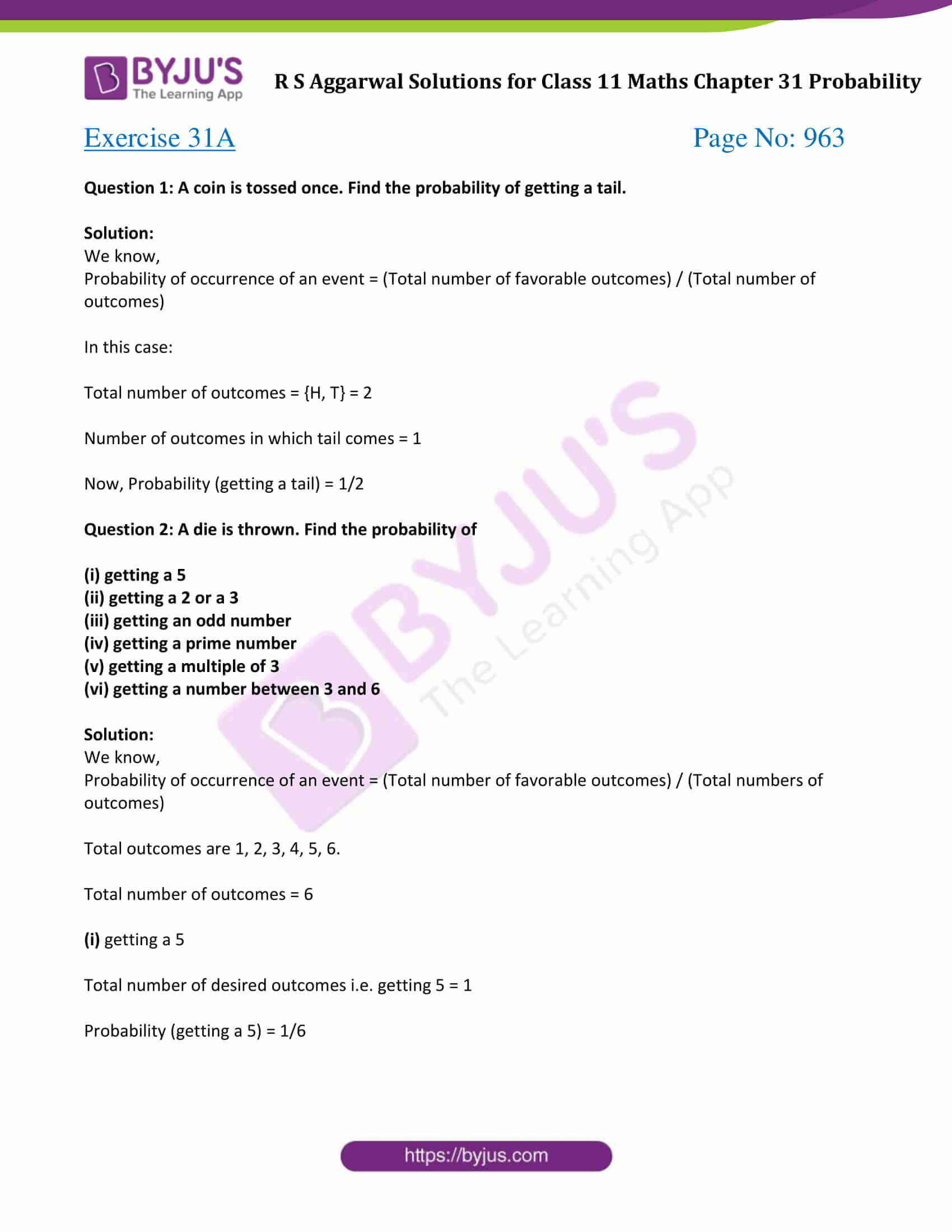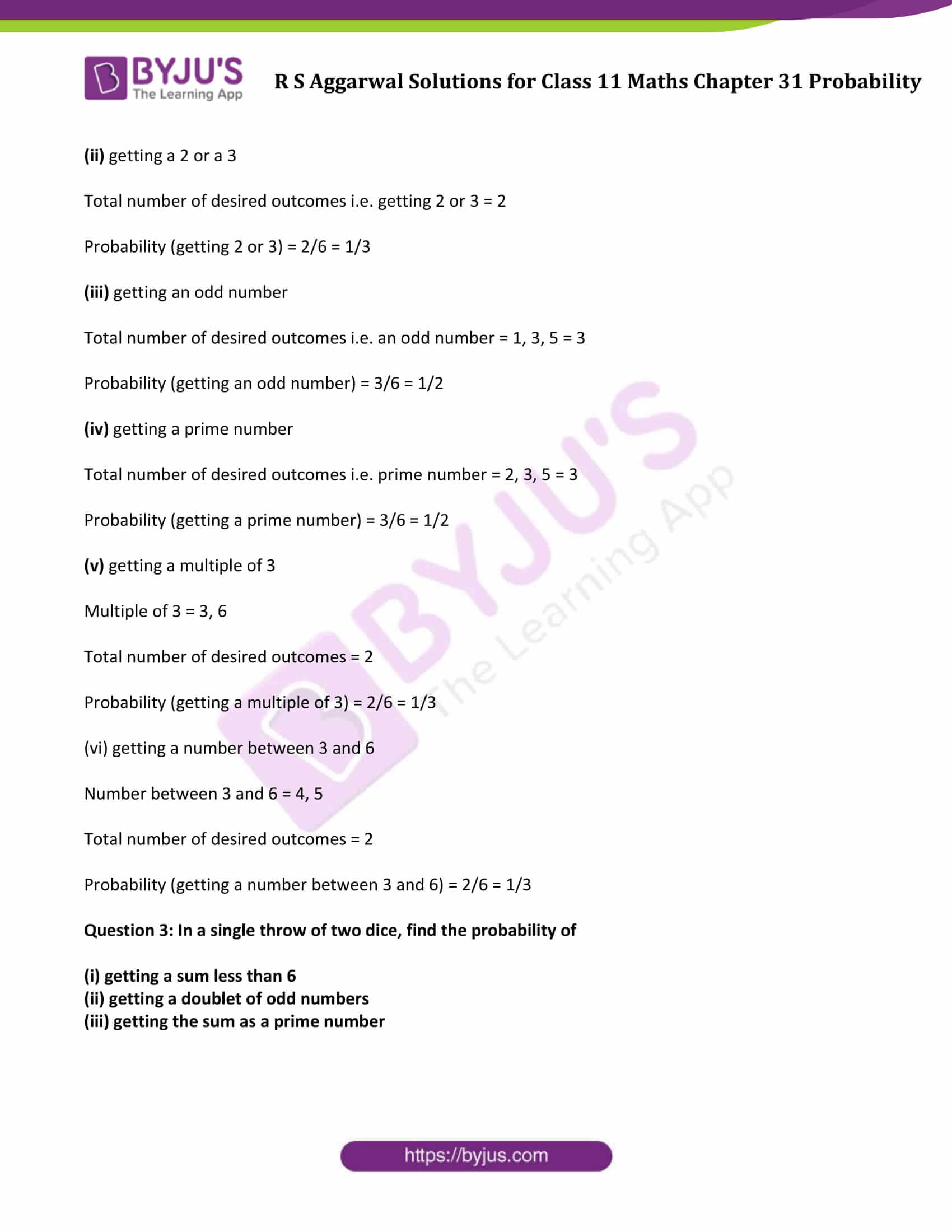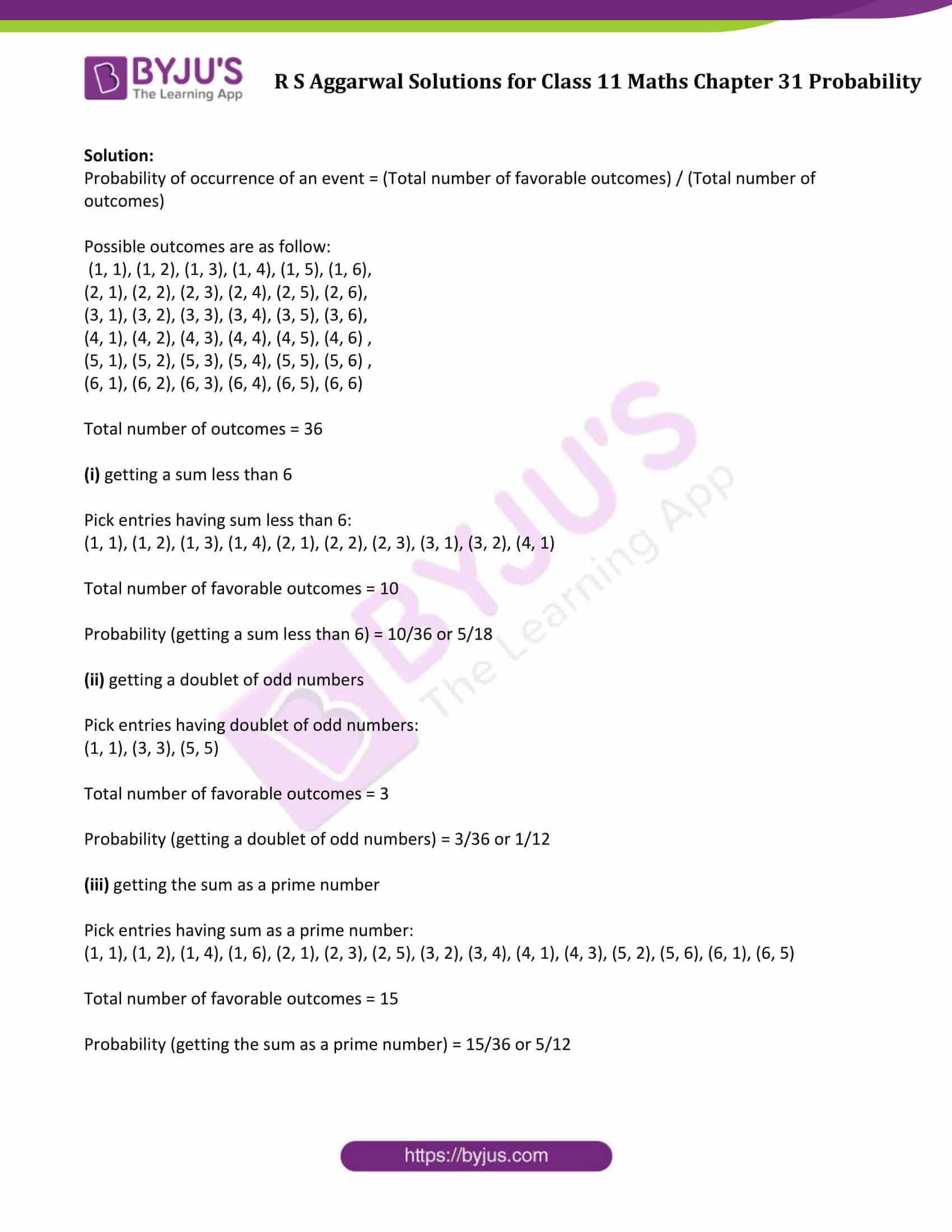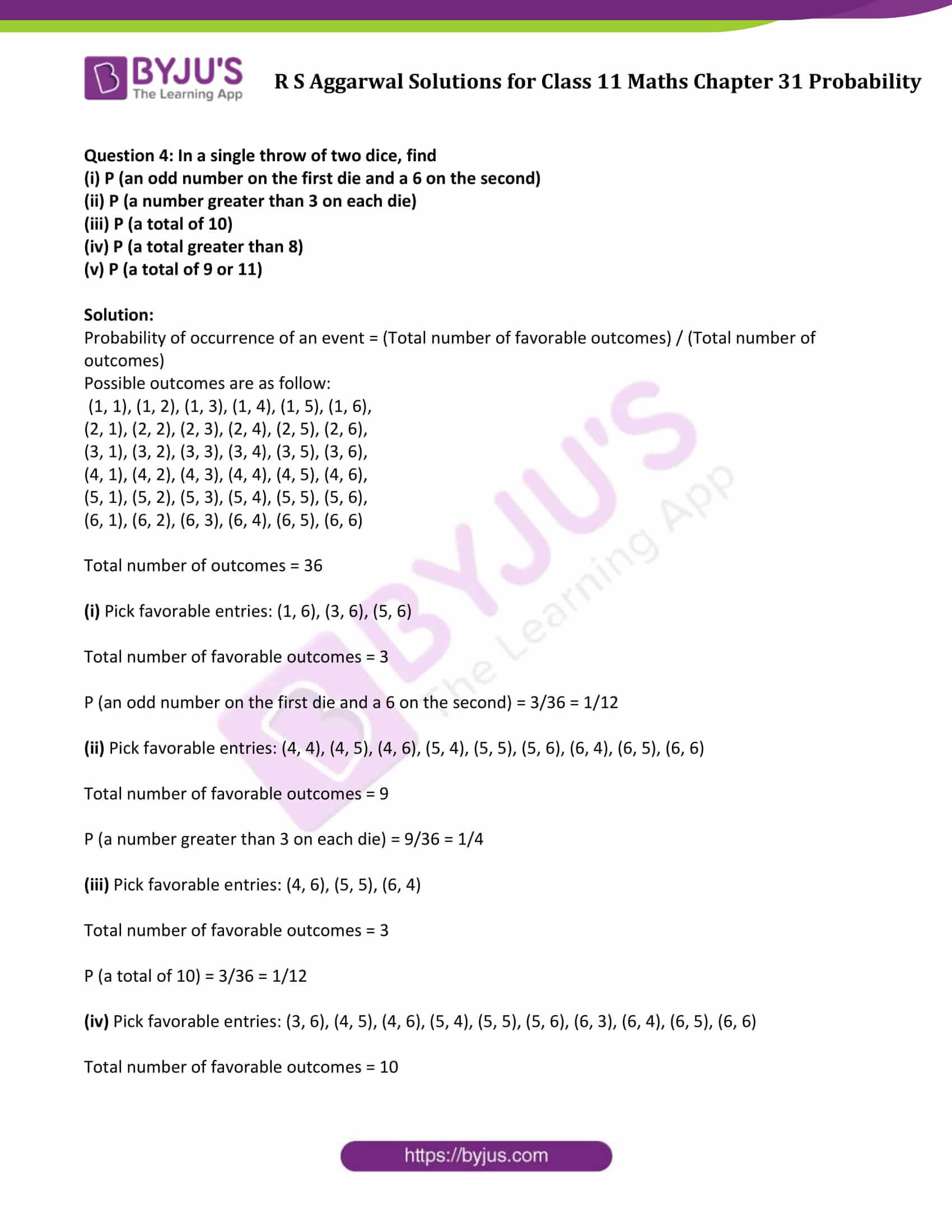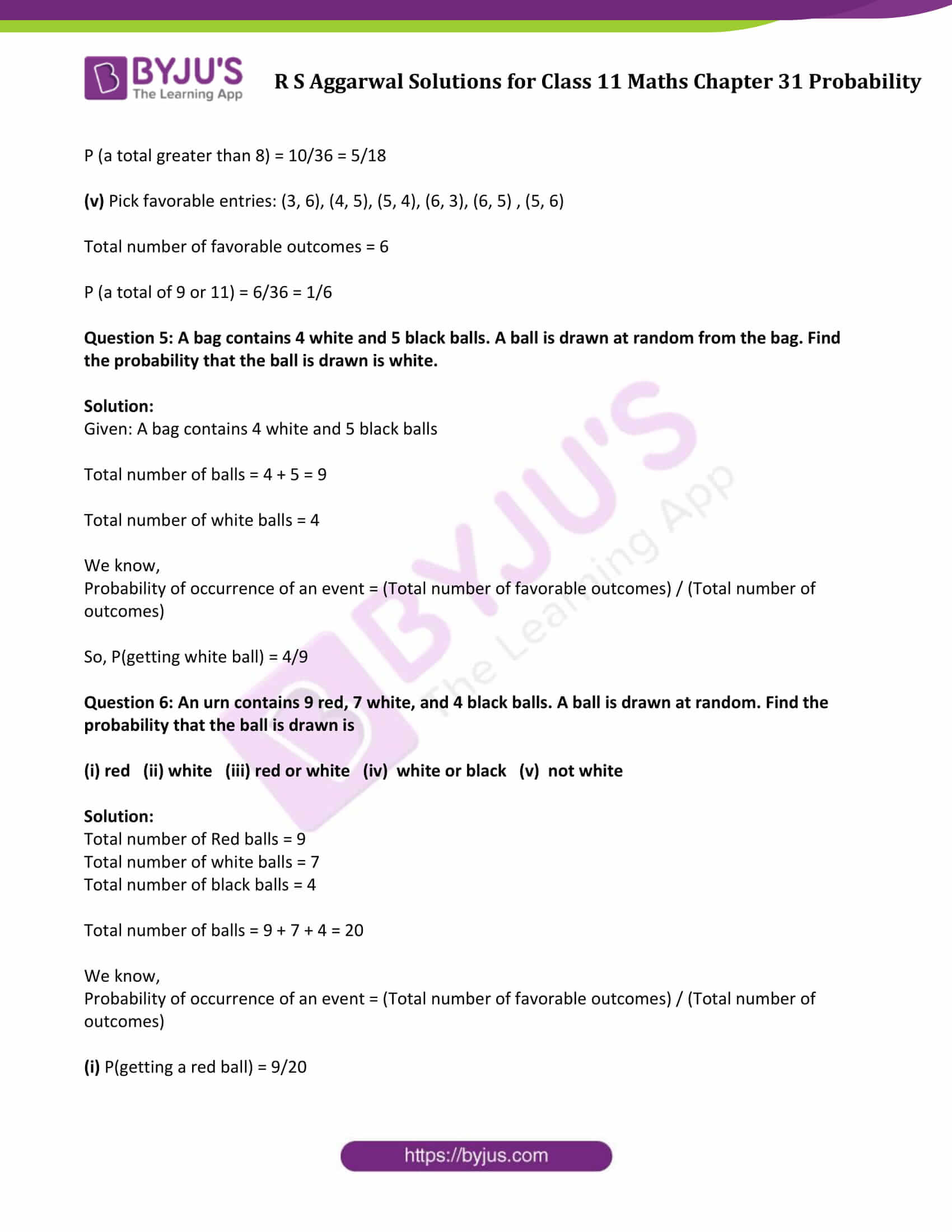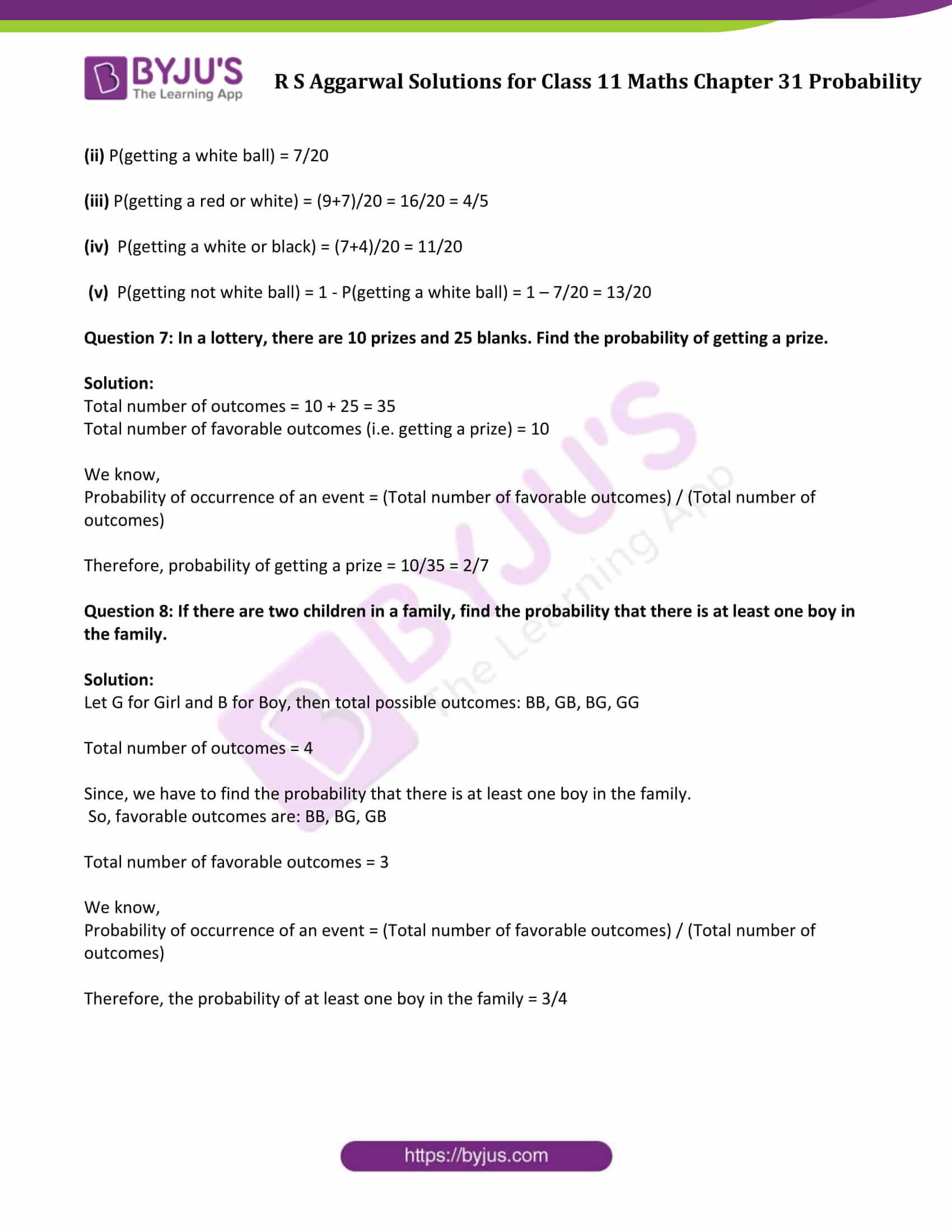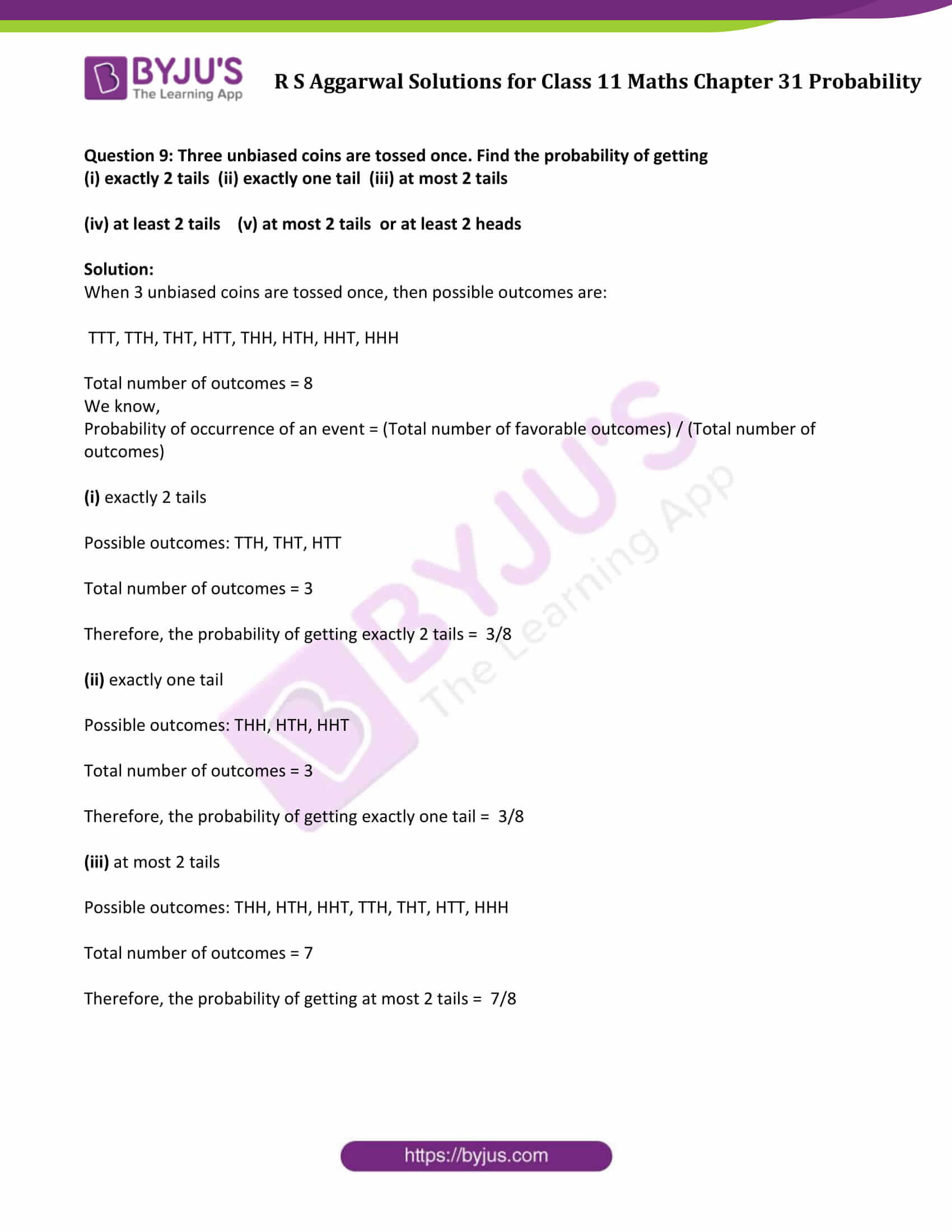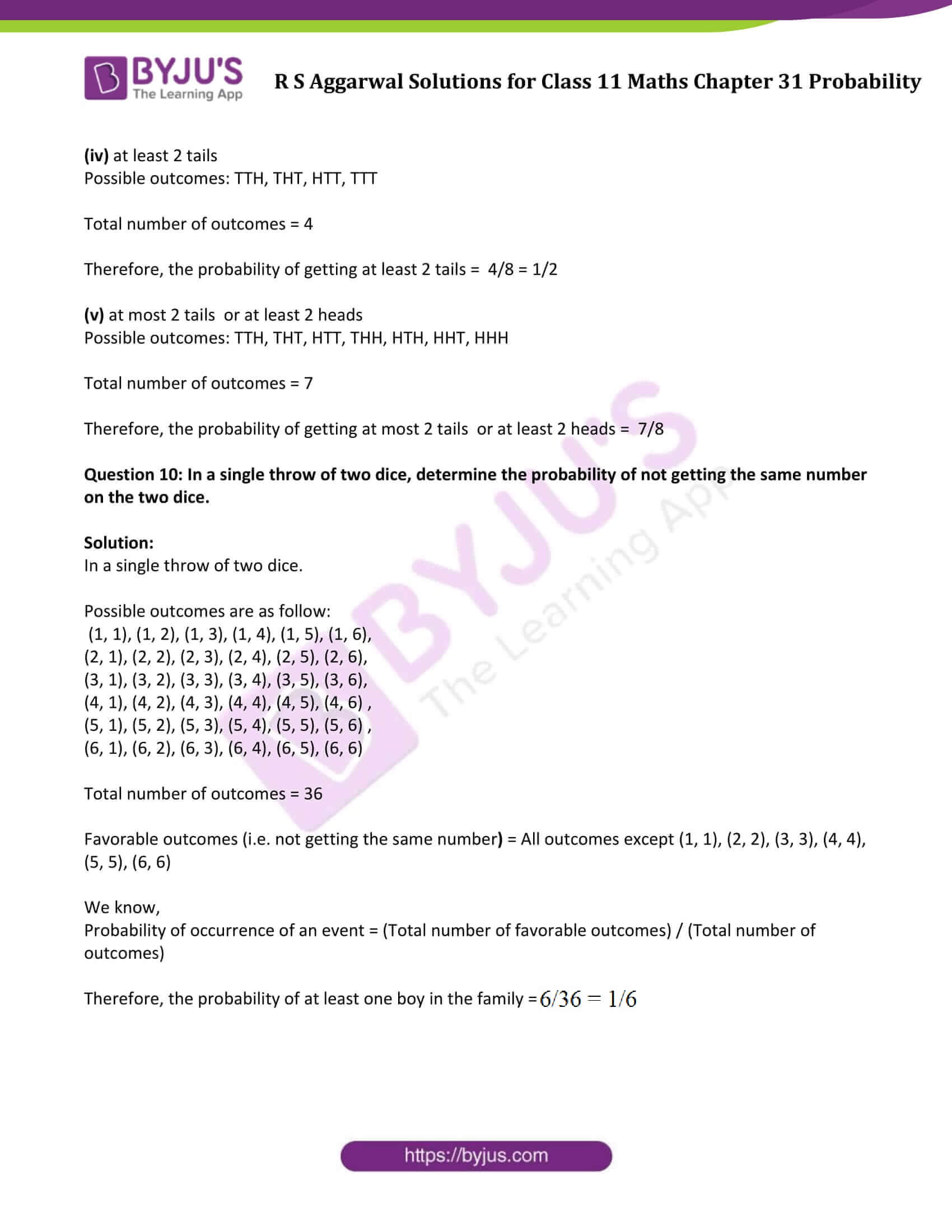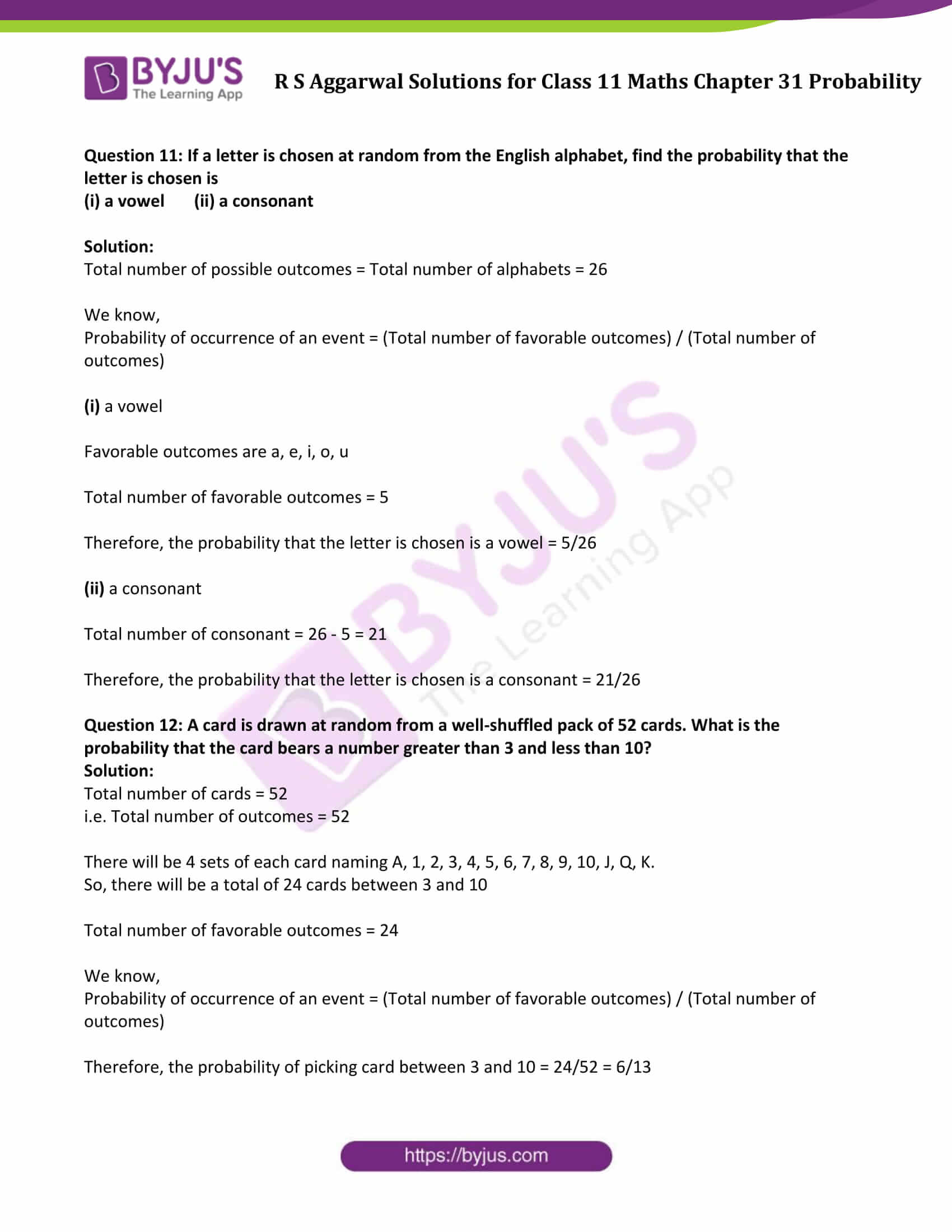### Access Answers to Maths R S Aggarwal Class 11 Chapter 31 Probability Exercise 31A Page number 963

Question 1: A coin is tossed once. Find the probability of getting a tail.

Solution:

We know,

Probability of occurrence of an event = (Total number of favorable outcomes) / (Total number of outcomes)

In this case:

Total number of outcomes = {H, T} = 2

Number of outcomes in which tail comes = 1

Now, Probability (getting a tail) = 1/2

Question 2: A die is thrown. Find the probability of

(i) getting a 5

(ii) getting a 2 or a 3

(iii) getting an odd number

(iv) getting a prime number

(v) getting a multiple of 3

(vi) getting a number between 3 and 6

Solution:

We know,

Probability of occurrence of an event = (Total number of favorable outcomes) / (Total number of outcomes)

Total outcomes are 1, 2, 3, 4, 5, 6.

Total number of outcomes = 6

(i) getting a 5

Total number of desired outcomes i.e. getting 5 = 1

Probability (getting a 5) = 1/6

(ii) getting a 2 or a 3

Total number of desired outcomes i.e. getting 2 or 3 = 2

Probability (getting 2 or 3) = 2/6 = 1/3

(iii) getting an odd number

Total number of desired outcomes i.e. an odd number = 1, 3, 5 = 3

Probability (getting an odd number) = 3/6 = 1/2

(iv) getting a prime number

Total number of desired outcomes i.e. prime number = 2, 3, 5 = 3

Probability (getting a prime number) = 3/6 = 1/2

(v) getting a multiple of 3

Multiple of 3 = 3, 6

Total number of desired outcomes = 2

Probability (getting a multiple of 3) = 2/6 = 1/3

(vi) getting a number between 3 and 6

Number between 3 and 6 = 4, 5

Total number of desired outcomes = 2

Probability (getting a number between 3 and 6) = 2/6 = 1/3

Question 3: In a single throw of two dice, find the probability of

(i) getting a sum less than 6

(ii) getting a doublet of odd numbers

(iii) getting the sum as a prime number

Solution:

Probability of occurrence of an event = (Total number of favorable outcomes) / (Total number of outcomes)

Possible outcomes are as follow:

(1, 1), (1, 2), (1, 3), (1, 4), (1, 5), (1, 6),

(2, 1), (2, 2), (2, 3), (2, 4), (2, 5), (2, 6),

(3, 1), (3, 2), (3, 3), (3, 4), (3, 5), (3, 6),

(4, 1), (4, 2), (4, 3), (4, 4), (4, 5), (4, 6) ,

(5, 1), (5, 2), (5, 3), (5, 4), (5, 5), (5, 6) ,

(6, 1), (6, 2), (6, 3), (6, 4), (6, 5), (6, 6)

Total number of outcomes = 36

(i) getting a sum less than 6

Pick entries having sum less than 6:

(1, 1), (1, 2), (1, 3), (1, 4), (2, 1), (2, 2), (2, 3), (3, 1), (3, 2), (4, 1)

Total number of favorable outcomes = 10

Probability (getting a sum less than 6) = 10/36 or 5/18

(ii) getting a doublet of odd numbers

Pick entries having doublet of odd numbers:

(1, 1), (3, 3), (5, 5)

Total number of favorable outcomes = 3

Probability (getting a doublet of odd numbers) = 3/36 or 1/12

(iii) getting the sum as a prime number

Pick entries having sum as a prime number:

(1, 1), (1, 2), (1, 4), (1, 6), (2, 1), (2, 3), (2, 5), (3, 2), (3, 4), (4, 1), (4, 3), (5, 2), (5, 6), (6, 1), (6, 5)

Total number of favorable outcomes = 15

Probability (getting the sum as a prime number) = 15/36 or 5/12

Question 4: In a single throw of two dice, find

(i) P (an odd number on the first die and a 6 on the second)

(ii) P (a number greater than 3 on each die)

(iii) P (a total of 10)

(iv) P (a total greater than 8)

(v) P (a total of 9 or 11)

Solution:

Probability of occurrence of an event = (Total number of favorable outcomes) / (Total number of outcomes)

Possible outcomes are as follow:

(1, 1), (1, 2), (1, 3), (1, 4), (1, 5), (1, 6),

(2, 1), (2, 2), (2, 3), (2, 4), (2, 5), (2, 6),

(3, 1), (3, 2), (3, 3), (3, 4), (3, 5), (3, 6),

(4, 1), (4, 2), (4, 3), (4, 4), (4, 5), (4, 6),

(5, 1), (5, 2), (5, 3), (5, 4), (5, 5), (5, 6),

(6, 1), (6, 2), (6, 3), (6, 4), (6, 5), (6, 6)

Total number of outcomes = 36

(i) Pick favorable entries: (1, 6), (3, 6), (5, 6)

Total number of favorable outcomes = 3

P (an odd number on the first die and a 6 on the second) = 3/36 = 1/12

(ii) Pick favorable entries: (4, 4), (4, 5), (4, 6), (5, 4), (5, 5), (5, 6), (6, 4), (6, 5), (6, 6)

Total number of favorable outcomes = 9

P (a number greater than 3 on each die) = 9/36 = 1/4

(iii) Pick favorable entries: (4, 6), (5, 5), (6, 4)

Total number of favorable outcomes = 3

P (a total of 10) = 3/36 = 1/12

(iv) Pick favorable entries: (3, 6), (4, 5), (4, 6), (5, 4), (5, 5), (5, 6), (6, 3), (6, 4), (6, 5), (6, 6)

Total number of favorable outcomes = 10

P (a total greater than 8) = 10/36 = 5/18

(v) Pick favorable entries: (3, 6), (4, 5), (5, 4), (6, 3), (6, 5) , (5, 6)

Total number of favorable outcomes = 6

P (a total of 9 or 11) = 6/36 = 1/6

Question 5: A bag contains 4 white and 5 black balls. A ball is drawn at random from the bag. Find the probability that the ball is drawn is white.

Solution:

Given: A bag contains 4 white and 5 black balls

Total number of balls = 4 + 5 = 9

Total number of white balls = 4

We know,

Probability of occurrence of an event = (Total number of favorable outcomes) / (Total number of outcomes)

So, P(getting white ball) = 4/9

Question 6: An urn contains 9 red, 7 white, and 4 black balls. A ball is drawn at random. Find the probability that the ball is drawn is

(i) red (ii) white (iii) red or white (iv) white or black (v) not white

Solution:

Total number of Red balls = 9

Total number of white balls = 7

Total number of black balls = 4

Total number of balls = 9 + 7 + 4 = 20

We know,

Probability of occurrence of an event = (Total number of favorable outcomes) / (Total number of outcomes)

(i) P(getting a red ball) = 9/20

(ii) P(getting a white ball) = 7/20

(iii) P(getting a red or white) = (9+7)/20 = 16/20 = 4/5

(iv) P(getting a white or black) = (7+4)/20 = 11/20

(v) P(getting not white ball) = 1 – P(getting a white ball) = 1 – 7/20 = 13/20

Question 7: In a lottery, there are 10 prizes and 25 blanks. Find the probability of getting a prize.

Solution:

Total number of outcomes = 10 + 25 = 35

Total number of favorable outcomes (i.e. getting a prize) = 10

We know,

Probability of occurrence of an event = (Total number of favorable outcomes) / (Total number of outcomes)

Therefore, probability of getting a prize = 10/35 = 2/7

Question 8: If there are two children in a family, find the probability that there is at least one boy in the family.

Solution:

Let G for Girl and B for Boy, then total possible outcomes: BB, GB, BG, GG

Total number of outcomes = 4

Since, we have to find the probability that there is at least one boy in the family.

So, favorable outcomes are: BB, BG, GB

Total number of favorable outcomes = 3

We know,

Probability of occurrence of an event = (Total number of favorable outcomes) / (Total number of outcomes)

Therefore, the probability of at least one boy in the family = 3/4

Question 9: Three unbiased coins are tossed once. Find the probability of getting

(i) exactly 2 tails (ii) exactly one tail (iii) at most 2 tails

(iv) at least 2 tails (v) at most 2 tails or at least 2 heads

Solution:

When 3 unbiased coins are tossed once, then possible outcomes are:

TTT, TTH, THT, HTT, THH, HTH, HHT, HHH

Total number of outcomes = 8

We know,

Probability of occurrence of an event = (Total number of favorable outcomes) / (Total number of outcomes)

(i) exactly 2 tails

Possible outcomes: TTH, THT, HTT

Total number of outcomes = 3

Therefore, the probability of getting exactly 2 tails = 3/8

(ii) exactly one tail

Possible outcomes: THH, HTH, HHT

Total number of outcomes = 3

Therefore, the probability of getting exactly one tail = 3/8

(iii) at most 2 tails

Possible outcomes: THH, HTH, HHT, TTH, THT, HTT, HHH

Total number of outcomes = 7

Therefore, the probability of getting at most 2 tails = 7/8

(iv) at least 2 tails

Possible outcomes: TTH, THT, HTT, TTT

Total number of outcomes = 4

Therefore, the probability of getting at least 2 tails = 4/8 = 1/2

(v) at most 2 tails or at least 2 heads

Possible outcomes: TTH, THT, HTT, THH, HTH, HHT, HHH

Total number of outcomes = 7

Therefore, the probability of getting at most 2 tails or at least 2 heads = 7/8

Question 10: In a single throw of two dice, determine the probability of not getting the same number on the two dice.

Solution:

In a single throw of two dice.

Possible outcomes are as follow:

(1, 1), (1, 2), (1, 3), (1, 4), (1, 5), (1, 6),

(2, 1), (2, 2), (2, 3), (2, 4), (2, 5), (2, 6),

(3, 1), (3, 2), (3, 3), (3, 4), (3, 5), (3, 6),

(4, 1), (4, 2), (4, 3), (4, 4), (4, 5), (4, 6) ,

(5, 1), (5, 2), (5, 3), (5, 4), (5, 5), (5, 6) ,

(6, 1), (6, 2), (6, 3), (6, 4), (6, 5), (6, 6)

Total number of outcomes = 36

Favorable outcomes (i.e. not getting the same number) = All outcomes except (1, 1), (2, 2), (3, 3), (4, 4), (5, 5), (6, 6)

We know,

Probability of occurrence of an event = (Total number of favorable outcomes) / (Total number of outcomes)

Therefore, the probability of at least one boy in the family = 6/36 = 1/6

Question 11: If a letter is chosen at random from the English alphabet, find the probability that the letter is chosen is

(i) a vowel (ii) a consonant

Solution:

Total number of possible outcomes = Total number of alphabets = 26

We know,

Probability of occurrence of an event = (Total number of favorable outcomes) / (Total number of outcomes)

(i) a vowel

Favorable outcomes are a, e, i, o, u

Total number of favorable outcomes = 5

Therefore, the probability that the letter is chosen is a vowel = 5/26

(ii) a consonant

Total number of consonant = 26 – 5 = 21

Therefore, the probability that the letter is chosen is a consonant = 21/26

Question 12: A card is drawn at random from a well-shuffled pack of 52 cards. What is the probability that the card bears a number greater than 3 and less than 10?

Solution:

Total number of cards = 52

i.e. Total number of outcomes = 52

There will be 4 sets of each card naming A, 1, 2, 3, 4, 5, 6, 7, 8, 9, 10, J, Q, K.

So, there will be a total of 24 cards between 3 and 10

Total number of favorable outcomes = 24

We know,

Probability of occurrence of an event = (Total number of favorable outcomes) / (Total number of outcomes)

Therefore, the probability of picking card between 3 and 10 = 24/52 = 6/13

## Access other exercise solutions of Class 11 Maths Chapter 31 Probability

Exercise 31B Solutions